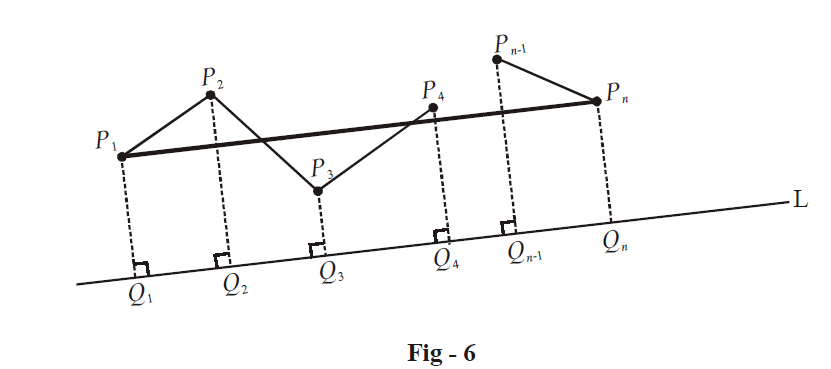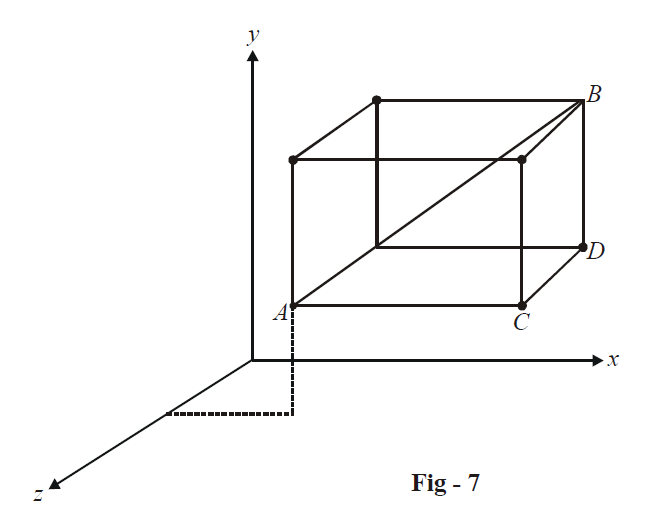# Examples on Direction Cosines and Ratios of Lines Set 1

Go back to  'Three Dimensional Geometry'

Example – 3

How many lines can we draw that are equally inclined to each of the three coordinate axis?

Solution: Intuitively, we can expect the answer to be 8, one for each of the 8 octants. Lets try to derive this answer rigorously.

Assume the direction cosines of the lines to be l, m, n. Thus,

${l^2} + {m^2} + {n^2} = 1\,\,\,\,\,\,\,\,\,\,\,\,\,\,\,\,\,\,\,\,\,\,\,\,\,\,\,\,\,\, \ldots \left( 1 \right)$

But since the lines are equally inclined to the three axes, we have   $$\left| l \right| = \left| m \right| = \left| n \right|.$$  This gives using (1),

\begin{align}&\qquad\;\;\;\left| l \right| = \left| m \right| = \left| n \right| = \frac{1}{{\sqrt 3 }}\\ &\Rightarrow\quad l = \pm \frac{1}{{\sqrt 3 }},\,\,\,m = \pm \frac{1}{{\sqrt 3 }},\,\,\,n = \pm \frac{1}{{\sqrt 3 }} \\ \end{align}

It is obvious that 8 combinations of l, m, n are possible. Hence, 8 lines can be drawn which are equally inclined to the axes.

Example – 4

Find the direction cosines of the line segment joining  $$A({x_1},\,{y_1},\,{z_1})$$  and $$B({x_2},\,{y_2},\,{z_2})$$

Solution: Refer to Fig - 4. Note that the x, y and z-components of the segment AB are AD, CB and DC respectively. If the direction cosines of AB are l, m, n and the length of AB is d, we have

$ld = {x_2} - {x_1},\,\,\,md = {y_2} - {y_1},\,\,nd = {z_2} - {z_1}$

Thus, the direction cosines of AB are given by

$\boxed{l = \frac{{{x_2} - {x_1}}}{d},\,\,\,m = \frac{{{y_2} - {y_1}}}{d},\,\,\,n = \frac{{{z_2} - {z_1}}}{d}}$

This result is quite important and will be used frequently in subsequent discussions.

Example – 5

Find the projection of the line segment joining the points  $$A({x_1},{y_1},{z_1})$$  and  $$B({x_2},\,{y_2},\,{z_2})$$  onto a line with direction cosines l, m, n.

Solution: Let us first consider a vector approach to this problem. The vector  $$\overrightarrow {AB}$$ can be written as

$\overrightarrow {AB} = \left( {{x_2} - {x_1}} \right)\hat i + \left( {{y_2} - {y_1}} \right)\hat j + \left( {{z_2} - {z_1}} \right)\hat k$

A unit vector  $$\hat{u}$$ along the line with direction cosines l, m, n will be

$\hat u = l\hat i + m\hat j + n\hat k$

Therefore, the projected length of  $$\overrightarrow {AB}$$  upon this line will be.

\begin{align}& d = \left| {\overrightarrow {AB} \cdot \hat u} \right| \\\\ \,\,\,\, &\;\;= \left| {l\left( {{x_2} - {x_1}} \right) + m\left( {{y_2} - {y_1}} \right) + n\left( {{z_2} - {z_1}} \right)} \right| \\\end{align}

This assertion can also be proved without resorting to the use of vectors. For this, we first understand the projection of a sequence of line segments on a given line.

Assume  $${P_1},{P_2},{P_3}.....{P_n}$$   to be n points in space. The sum of projections of the sequence of segments  $${P_1}{P_2},{P_2}{P_3},.....{P_{n - 1}}{P_n}$$  onto a fixed line L will be the same as the projection of  $${P_1}{P_n}$$ onto L. This should be obvious from the following diagram:The projection of the segment  $${P_1}{P_n}$$  onto L is  $${Q_1}{Q_n}.$$  The sum of projections of segments  $${P_1}{P_2},{P_2}{P_3}.....{P_{n - 1}}{P_n}$$  onto L is  $${Q_1}{Q_2} + {Q_2}{Q_3} + ..... + {Q_{n - 1}}{Q_n} = {Q_1}{Q_n}.$$

We use this fact in our original problem as follows:The projection d of AB onto any line L (with direction cosines say l, m, n) will be sum of projections of AC, CD, DB onto L. Since AC, CD, and DB are  $$l\left( {{x_2} - {x_1}} \right),\,m\left( {{y_2} - {y_1}} \right)$$  and  $$n\left( {{z_2} - {z_1}} \right)$$  respectively, we get the total projection of AB onto L as

$d = \left| {l\left( {{x_2} - {x_1}} \right) + m\left( {{y_2} - {y_1}} \right) + n\left( {{z_2} - {z_1}} \right)} \right|$

Learn from the best math teachers and top your exams

• Live one on one classroom and doubt clearing
• Practice worksheets in and after class for conceptual clarity
• Personalized curriculum to keep up with school